Class IX Science

# Chapter - 12 Sound

## NCERT Solutions for Class 9 Science

Other Chapters
In page question - Page 162
Q 1.

How does the sound produced by a vibrating object in a medium reach your ear?

Ans.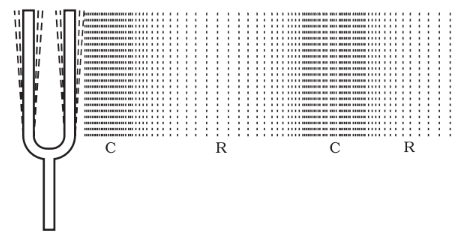When a body vibrates the air in its neighborhood is alternately compressed and rarefied. The compressed air has higher pressure than surrounding air. It therefore pushes the air particles near it causing compression to move forward. A rarefaction or low pressure is created at the original place. These compressions and rarefaction causes particles in the air to vibrate about their mean position. The energy is carried forward in these vibration. This is how sound travels.

In page question - Page 163
Q 1.

Explain how sound is produced by your school bell?

Ans.

When the gong strikes the bell, vibrations are produced in the bell which are transmitted through the air to our ears. These vibration produce sensation of sound in our ears.

In page question - Page 163
Q 2.

Why are sound waves called mechanical waves?

Ans.

Sound waves are called mechanical waves because they need a material medium to travel.

In page question - Page 163
Q 3.

Suppose you and your friend are on the moon. Will you be able to hear any sound produced by your friend?

Ans.

On the moon, sound cannot travel as there is no atmosphere. Sound cannot travel in vacuum so we will not be able to hear any sound.

In page question - Page 166
Q 1.

Which waves property determines

1. loudness
2. pitch?
Ans.
1. Loudness is determined by the amplitude of the sound. Greater the amplitude more will be the loudness.
2. Pitch is determined by frequency. Higher is the frequency, greater will be the pitch.
In page question - Page 166
Q 2.

Guess which sound has a higher pitch: guitar or car horn?

Ans.

Guitar

In page question - Page 166
Q 3.

What are wavelength, frequency, time period and amplitude of a sound wave?

Ans.

Wavelength: The distances between two consecutive compressions or rarefaction of a wave. Its S.I unit is meter.

Frequency: One compression and one rarefaction constitutes one vibration. The number of vibration in a second is called frequency. Its unit is Hertz.

Amplitude: When waves are produced, the particles vibrate about their mean position. The maximum displacement from its mean position of a particle is called its amplitude. It is measured in meters.

Time period: The time taken by the wave to complete one oscillation i.e., the time between two consecutive compressions or rarefactions is called time period.

In page question - Page 166
Q 4.

How are the wavelength and frequency of a sound wave related to its speed?

Ans.

Speed = Wavelength x frequency
V = λ × ν

In page question - Page 166
Q 5.

Calculate the wavelength of a sound wave whose frequency is 220 Hz and speed is 440 m/s in a given medium.

Ans.

f = 220 Hz, V = 440 m/s

λ =

In page question - Page 166
Q 6.

A person is listening to a tone of 500 Hz sitting at a distance of 450 m from the source of the sound. What is the time interval between successive compressions from the source?

Ans.

ν = 500 Hz.

Therefore T =

In page question - Page 166
Q 7.

Distinguish between loudness and intensity of sound?

Ans.

Loudness and intensity both depend upon the amplitude of sound. But loudness is the physiological response of our ears to a particular frequency. Our ears are more sensitive to some frequencies as compared to others. Intensity is the amount of sound energy passing per second per unit area. It is proportional to square of amplitude.

In page question - Page 167
Q 1.

In which of the three media, air, water or iron, does sound travel the fastest at a particular temperature?

Ans.

Sound travels faster in iron and slowest in air.

In page question - Page 168
Q 1.

An echo returned in 3 s. What is the distance of the reflecting surface from the source, given that the speed of sound is 342 m/s?

Ans.

Time for echo = 3 s

v = 342 m/s
Therefore distance: d = v × t = 342×3 m = 513 m.

In page question - Page 169
Q 1.

Why are the ceilings of concert halls curved?

Ans.

The ceilings of concert halls are curved so that after reflections from the surface, sound can reach each and every part of the hall.

In page question - Page 170
Q 1.

What is the audible range of the average human ear?

Ans.

Audible range 20 Hz − 20,000 Hz

In page question - Page 170
Q 2.

What is the range of frequencies associated with

1. Infra sound?
2. Ultrasound?
Ans.
1. Infra-sound less than 20 Hz
2. Ultra-sound greater than 20, 000 Hz.
In page question - Page 172
Q 1.

A submarine emits a sonar pulse, which returns from an underwater cliff in 1.02 s. If the speed of sound in salt water is 1531 m/s, how far away is the cliff?

Ans.

t = 1.02 s, v = 1531 m/s
distance d = v × t = 1531×1.022 m = 780.81 m

Chapter end exercise
Q 1.

What is sound and how is it produced?

Ans.

Sound is a form of energy and it is produced due to vibrations of different types of object.

For example: A vibrating tuning fork, a bell, wires in a sitar and a guitar etc.

Chapter end exercise
Q 2.

Describe with the help of a diagram, how compressions and rarefactions are produced in air near a source of sound.

Ans.

If we blow a horn, speak, or produce sound by an object in air we are pushing the air molecules. These molecules, in turn, push the adjacent molecules which impart their energy to the next ones. After losing energy in the interaction, the molecule is back to its original (mean position. This results in formation of compressions and rarefactions.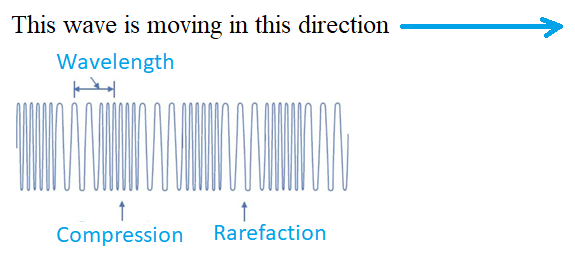Chapter end exercise
Q 3.

Cite an experiment to show that sound needs a material medium for its propagation.

Ans.

Take an electric bell and an airtight glass bell jar. The electric bell is suspended inside the airtight bell jar. The bell jar is connected to a vacuum pump, as shown in following figure. Now, if we press the switch, we will be able to hear the bell.

Now start the vacuum pump. When the air in the jar is pumped out gradually, the sound becomes fainter, although the same current is passing through the bell.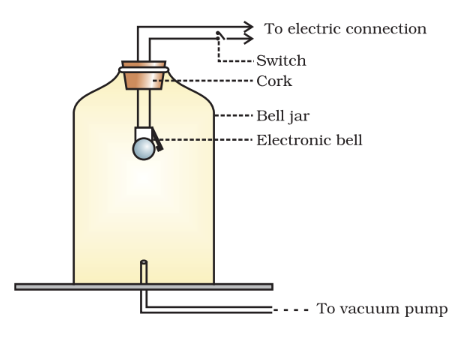After some time when less air is left inside the bell jar you will hear a very feeble sound. At the end, when all the air is removed completely, we will not be able to hear the sound of bell. This shows sound requires material medium.

Chapter end exercise
Q 4.

Why sound wave is called a longitudinal wave?

Ans.

A sound wave is called a longitudinal wave because it travels in the form of compressions and  rarefactions in the medium, where the particles of the medium vibrate in a direction which is parallel to the direction of propagation of the sound wave.

Chapter end exercise
Q 5.

Which characteristic of the sound helps you to identify your friend by his voice while sitting with others in a dark room?

Ans.

The timbre of sound is that characteristic which enables us to distinguish one sound from the other even when these are of the same pitch and loudness. Each person has its own timbre of sound and this characteristic helps us to identify a person from others even without looking at him (i.e., in a dark room).

Chapter end exercise
Q 6.

Flash and thunder are produced simultaneously. But thunder is heard a few seconds after the flash is seen, why?

Ans.

The flash of light is seen earlier than the thunder of sound even though both are produced simultaneously because the speed of light (c) is greater than speed of sound (v) by 106 as $\frac{c}{v}$

Chapter end exercise
Q 7.

A person has a hearing range from 20 Hz to 20 kHz. What are the typical wavelengths of sound waves in air corresponding to these two frequencies? Take the speed of sound in air as 344 m/s

Ans.

Given,    v1  =  20 Hz

v2= 20 kHz = 20 x 10 3 Hz, and

Speed of sound, v= 344 m/s

Clearly,

Chapter end exercise
Q 8.

Two children are at opposite ends of an aluminium rod. One strikes the end of the rod with a stone. Find the ratio of times taken by the sound wave in air and in aluminium to reach the second child.

Ans.

Speed of sound in air = 346 m/s and

Speed of sound in aluminium = 6420 m/s

Since time taken by sound to travel a given distance in a medium is inversely proportional to its speed in that medium,

Chapter end exercise
Q 9.

The frequency of a source of sound is 100 Hz. How many times does it vibrate in a minute?

Ans.

Since the frequency of the source of sound is 100 Hz,

Number of vibrations of the source in I second = 100

Number of vibrations of the source in I minute (i.e., 60 second) = 6000

Chapter end exercise
Q 10.

Does sound follow the same laws of reflection as light does? Explain.

Ans.

Yes, sound follow the same laws of reflection as light does.

The laws of reflection of sound are as follows:

1. The incident sound wave, the reflected sound wave, and the normal at the point of incident all lie in the plane.
2. The angle of incidence of sound wave and angle of reflection of sound wave to the normal are equal.
Chapter end exercise
Q 11.

When a sound is reflected from a distant object, an echo is produced. Let the distance between the reflecting surface and the source of sound production remains the same. Do you hear echo sound on a hotter day?

Ans.

In any medium as we increase the temperature the speed of sound increases. For example, the speed of sound in air is 331 m/s at 0oc and 344 m/s at 22oc. So, on a hotter day, we cannot here the echo between the same distances.

Chapter end exercise
Q 12.

Give two practical applications of reflection of sound waves.

Ans.

The practical applications of sound are as follows:

• Megaphones or loudhailers are based on multiple reflections of sound.
• Stethoscope is a medical instrument used for listening to sounds produced within the body is also based on multiple reflection of sound.
Chapter end exercise
Q 13.

A stone is dropped from the top of a tower 500 m high into a pond of water at the base of the tower. When is the splash heard at the top? Given, g = 10 m/s2 and speed of sound = 340 m/s

Ans.

During the downward motion of stone

Initial velocity       u = 0 m/s

Height                    h = 500 m

Acceleration           g = 10 m/s2

using the equation,

t = 100,

t =10

So, stone takes 10 seconds to pond from the top of the tower. Now a sound of splash is produced.

Now the time taken by the sound from base of tower to top of tower is given by

So, the total time taken = 10 seconds + 1.47 seconds = 11.47 seconds

Chapter end exercise
Q 14.

A sound wave travels at a speed of 339 m/s . If its wavelength is 1.5 cm, what is the frequency of the wave? Will it be audible?

Ans.

We know that, Frequency = Speed/Wavelength

Here, Speed = 339 m/s

Wavelength = 1.5 cm = 0.015 m

Therefore,

Frequency = 339/0.015 = 22600 Hz

It is not audible (as the audible frequency is 20 Hz to 20000 Hz).

Chapter end exercise
Q 15.

What is reverberation? How can it be reduced?

Ans.

A sound created in a big hall will persist by repeated reflection from the walls until it is reduced to a value where it is no longer audible. The repeated reflection that results in this persistence of sound is called reverberation.

To reduce reverberation, the roof and walls of the auditorium are generally covered with sound-absorbent materials like compressed fibreboard, rough plaster or draperies. The seat materials are also selected on the basis of their sound absorbing properties.

Chapter end exercise
Q 16.

What is loudness of sound? What factors does it depend on?

Ans.

The loudness (or softness) of a sound is determined by its amplitude. If amplitude is higher, it is a louder sound. It depends upon the force with which an object is made to vibrate.

Chapter end exercise
Q 17.

Explain how bats use ultrasound to catch a prey.

Ans.

Bats can produce and hear sound of frequency up to 100 kHz. The sound produced by flying bat gets reflected from its prey in front of it. By hearing this reflected sound, it can detect the prey even during nights.

Chapter end exercise
Q 18.

How is ultrasound used for cleaning?

Ans.

To clean any objects, it is placed in a cleaning solution and ultrasonic waves are sent into the solution. Due to the high frequency, the particles of dust, grease and dirt get detached and drop out. The objects thus get thoroughly cleaned.

Chapter end exercise
Q 20.

Explain the working and application of a sonar.

Ans.

Sonar consists of a transmitter and a detector and is installed in a boat or a ship, as shown in following figure.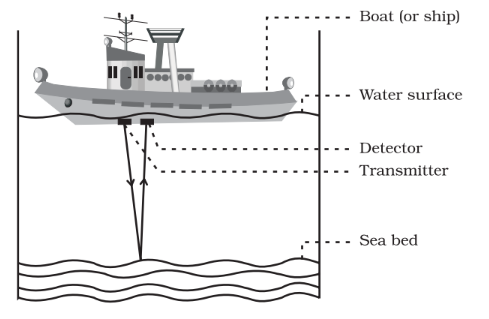The transmitter produces and transmits ultrasonic waves. These waves travel through water and after striking the object on the seabed, get reflected back and are sensed by the detector. The detector converts the ultrasonic waves into electrical signals which are appropriately interpreted. The distance of the object that reflected the sound wave can be calculated by knowing the speed of sound in water and the time interval between transmission and reception of the ultrasound. If the time interval between transmission and reception of ultrasound signal be t and the speed of sound through seawater be v. The total distance, 2d travelled by the ultrasound is then, 2d = v x t.

The sonar technique is used to determine the depth of the sea and to locate underwater hills, valleys, submarine, icebergs, sunken ship etc.

Chapter end exercise
Q 20.

A sonar device on a submarine sends out a signal and receives an echo 5 s later. Calculate the speed of sound in water if the distance of the object from the submarine is 3625 m.

Ans.

Total distance travelled by sound = 2 x 3625 = 7250 m

Time taken = 5 seconds

Therefore, speed = distance / time

= 7250 / 5 m/s

= 1450 m/s

So, the speed of sound in water is 1450 m/s.

Chapter end exercise
Q 21.

Explain how defects in a metal block can be detected using ultrasound.

Ans.

Ultrasounds can be used to detect the defect in metal blocks. The cracks or holes inside the metal blocks, which are invisible from outside reduces the strength of the structure. Ultrasonic waves are allowed to pass through the metal block and detectors are used to detect the transmitted waves. If there is even a small defect, the ultrasound gets reflected back indicating the presence of the flaw or defect, as shown in following figure.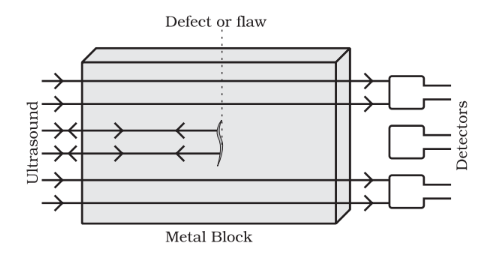Chapter end exercise
Q 22.

Explain how the human ear works.

Ans.

The outer ear is called 'pinna'. It collects the sound from the surroundings. The collected sound passes through the auditory canal. At the end of the auditory canal there is a thin membrane called the ear drum or tympanic membrane. When a compression of the medium reaches the eardrum the pressure on the outside of the membrane increases and forces the eardrum inward.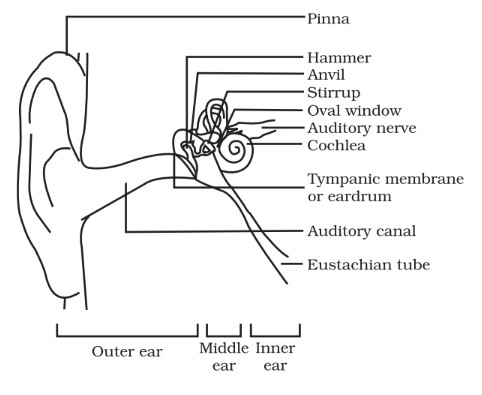Similarly, the eardrum moves outward when a rarefaction reaches it. In this way the eardrum vibrates. The vibrations are amplified several times by three bones (the hammer, anvil and stirrup) in the middle ear. The middle ear transmits the amplified pressure variations received from the sound wave to the inner ear. In the inner ear, the pressure variations are turned into electrical signals by the cochlea. These electrical signals are sent to the brain via the auditory nerve, and the brain interprets them as sound.